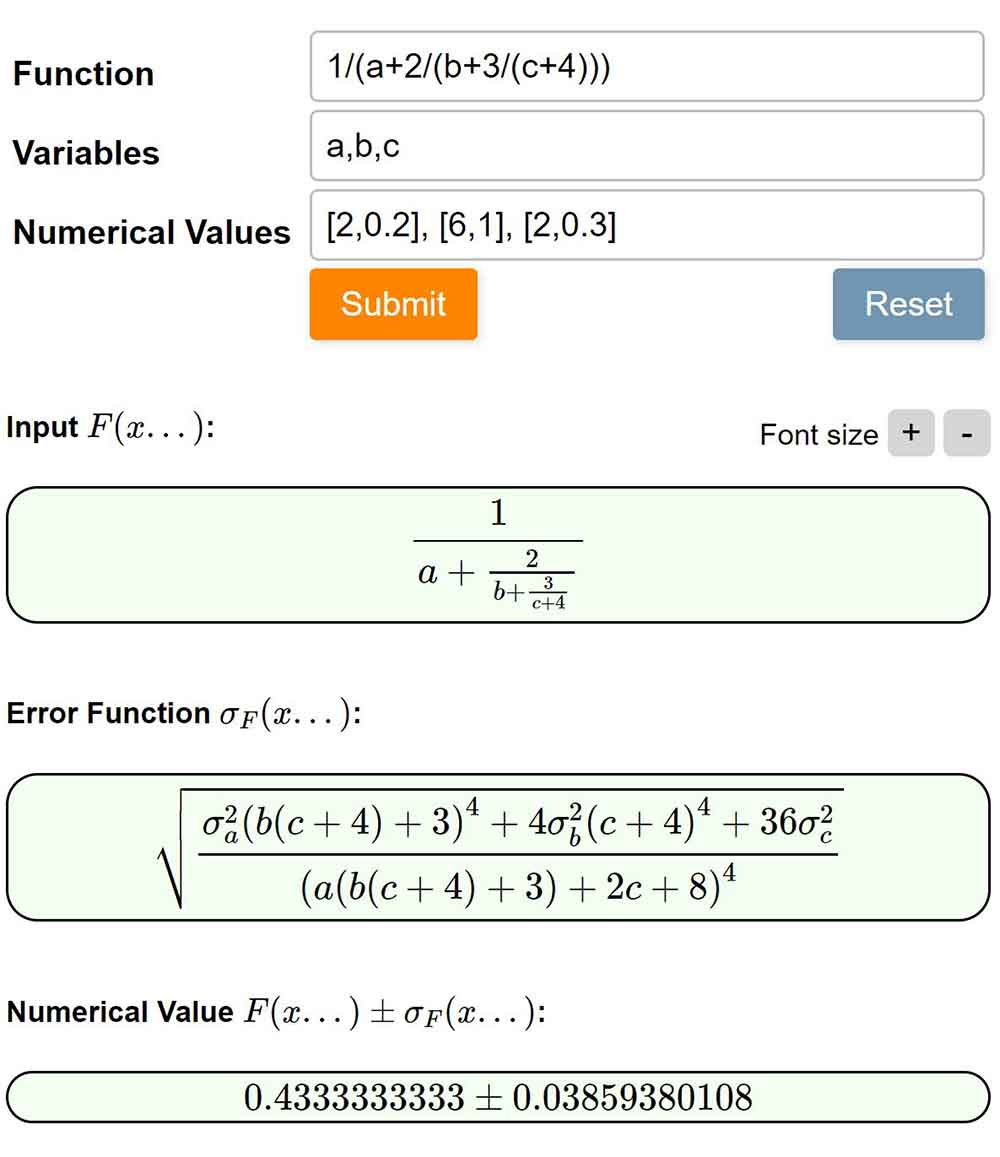## Error Propagation CalculatorThis application calculates error (uncertainty) propagation for any given arbitrary analytical function. It derives an analytical expression of the error propagation relation. It can also calculate numerical value of the function and its error if values are provided for input variables. See a demo here.

• The application derives full analytical form of the error propagation relation. Symbolic calculations are done using SymPy library.
• You may optionally specify the list of symbols which are to be treated of variable on which partial derivative are to be calculated. Other unused symbols will be treated as constants.
• You may also optionally assign value and error for each variables, which will be used to do numerical evaluation of the function and its error. The result can be mix of number and symbols, if there are constant symbols and/or numerical values are unassigned for some variables.
• All function and values are rendered as LaTeX and LaTeX commands can be copied by right clicking on it.
• If you have a complex function and the LaTeX rendering is too small, you can change font size using +/- buttons.

You may either use the tool from below or open it in an external window, from there you can share your calculation by copying the address bar URL.

Found a bug? report here.

## Changelog

Oct 02 2022:

• The backend computation engine has been moved from self-hosted server to so-called serverless application. This increased the speed by about 100%, but now the application timeout is set to 10 sec.
• Added option to disable simplification of analytical expressions. This will result into faster computation and also help to avoid timeouts for complex equations.

July 11 2021:

• In the output now log() will be represented as ln(). However, input format remains same, i.e, ln() and log() are natural logarithms, while log10() and lg() are logarithms of base 10.

June 28 2021:
Major update in equation parser to allow more natural input: please report if a equation is misinterpreted.

• Two symbols separated by space are interpreted as multiplication instead of throwing error. E.g, 'a b' -> 'a*b'
• number followed by a symbol name is treated as multiplication. E.g., 2alpha -> 2*alpha
• symbols adjacent to parenthesis are treated as multiplication. E.g., a(b-x) -> a*(b-x); (b-x)a -> (b-x)*a; 2(b-x) -> 2*(b-x)
• symbol lambda will be replaced as lamda for proper latex rendering.
• Numerical value input is now more flexible: comma and square brackets are not necessary. Separated sequence of numbers are treated as value error pairs. E.g., for three variables the value-error pairs can be like 2 0.1 5 0.2 7 0.3 . However, it is advised to maintain the old format with square bracket and comma, for clarity

June 15 2021:

• The variable list (either from input or auto determined) are shows as input arguments for F(...) and sigmaF(...) when Latex representing the output.